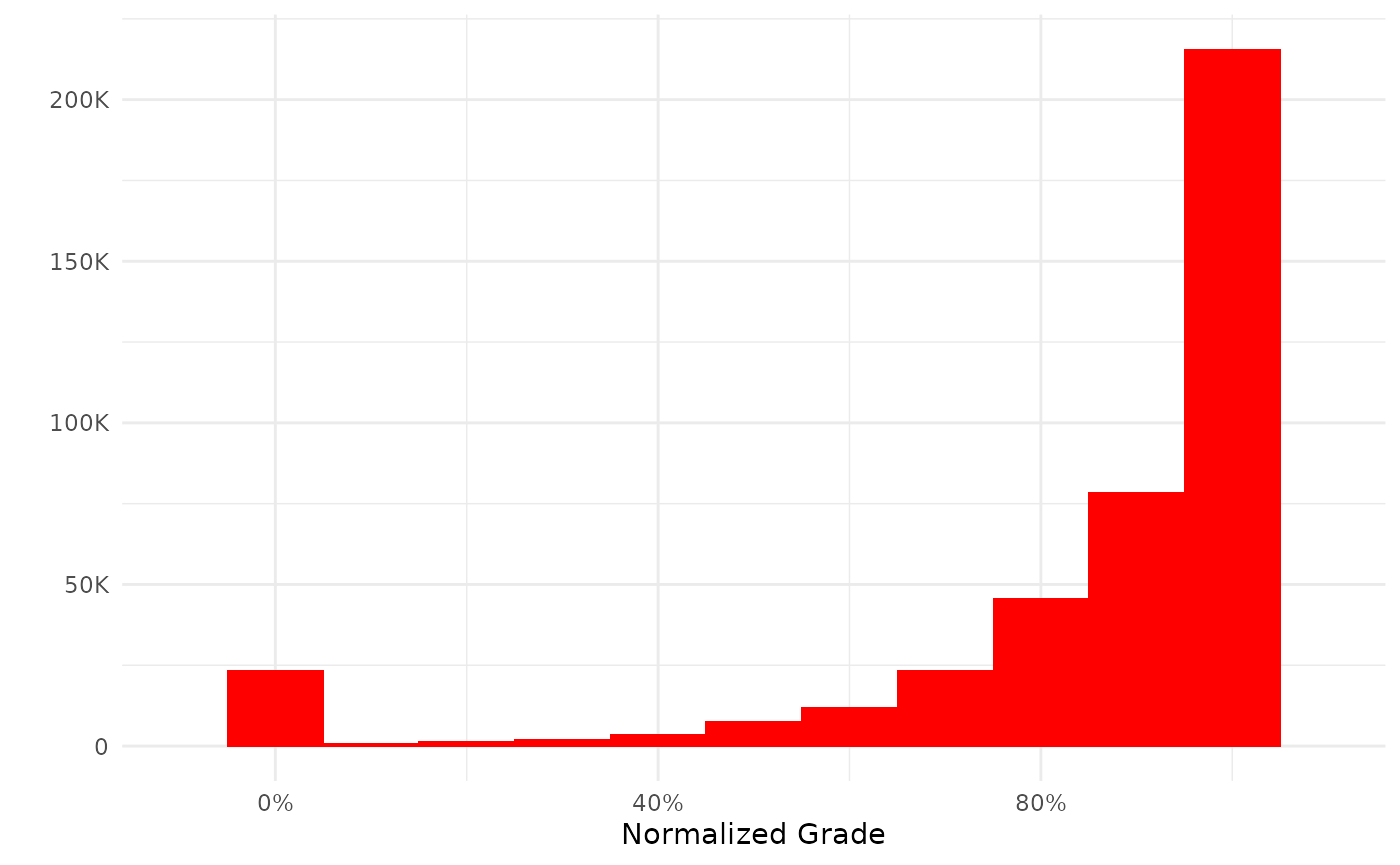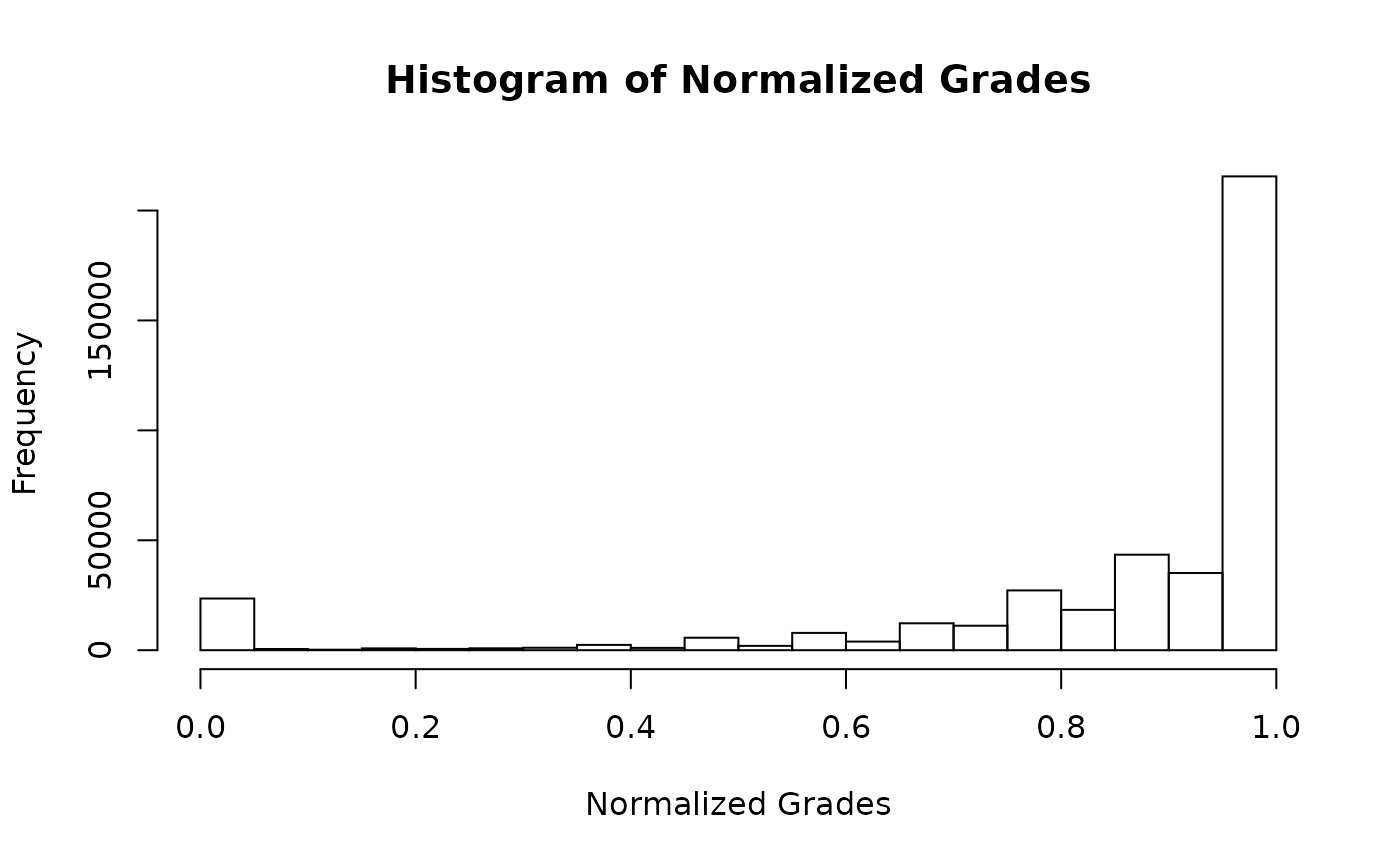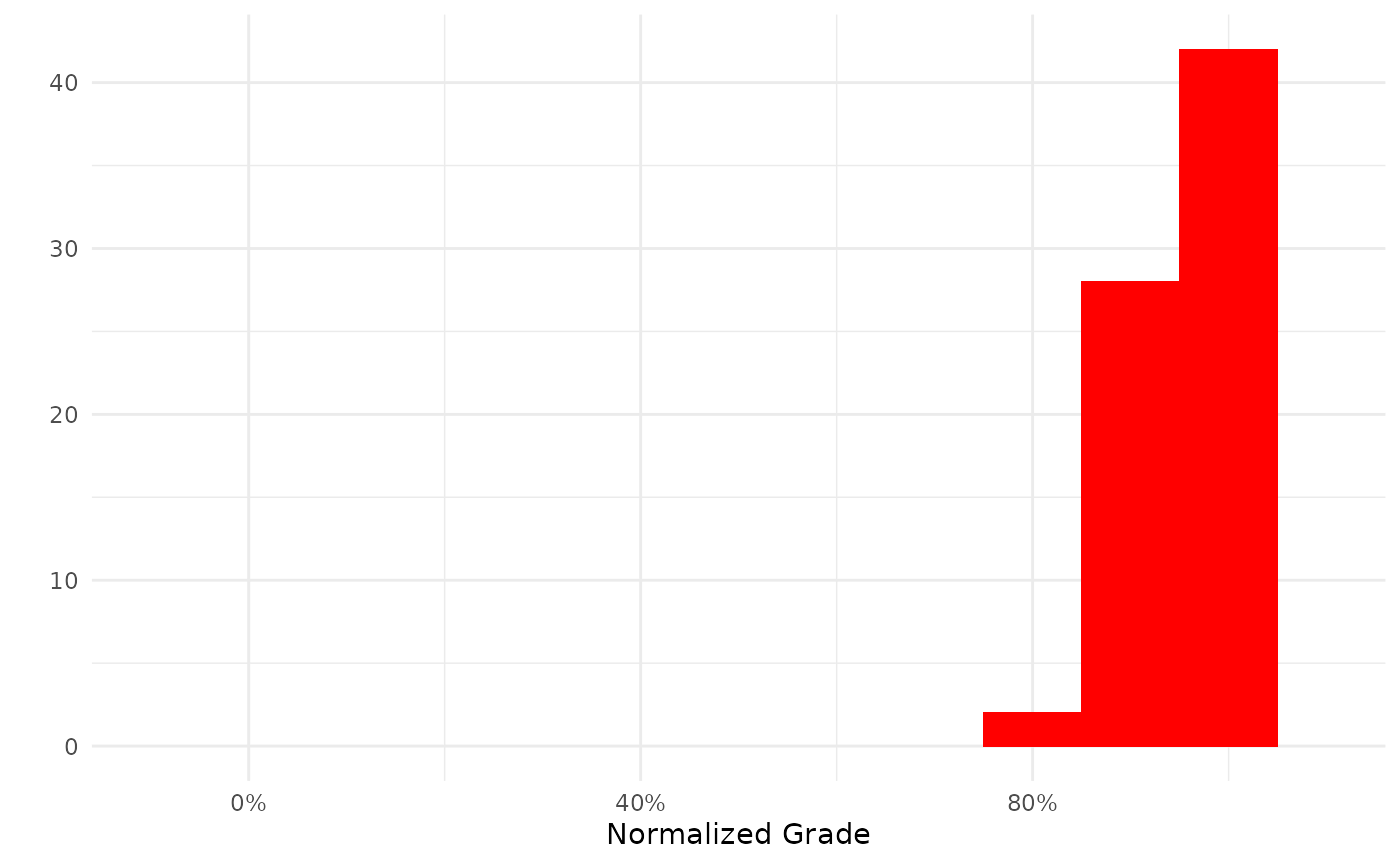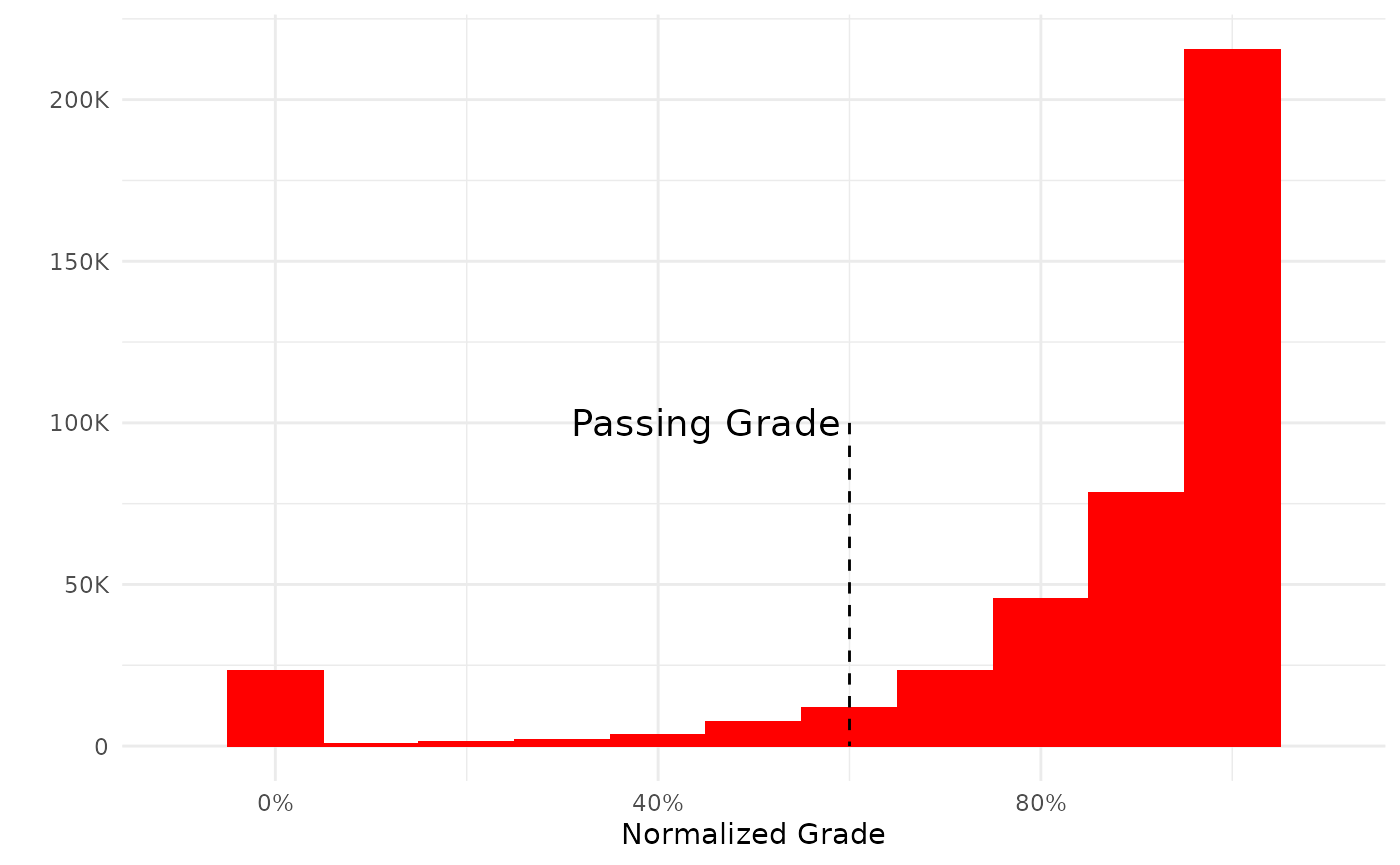This vignette assumes that you have already been able to set up the connection to a Moodle Database and created a local cache (if chosen).

library(moodleR)
library(ggplot2)

## Data access functions

Learning analytics practitioners working with LMS data will often be interested in a sub-set of the available tables with relevant references added. These can be accessed through several mdl_* functions which will connect to the relevant table(s) and return a reference to the data. Currently the package supports curated access through the following functions:

• mdl_courses()
• mdl_forum_posts()
• mdl_grades()
• mdl_log()
• mdl_users()

For example:

my_grades <- mdl_grades()

creates an R object named my_grades containing the dbplyr reference to the table in question.

## Summary functions

The R generic summary function is implemented with sensible defaults so that:

summary(my_grades)
#> ----------
#> Missing:      945.2K
#> Courses:      2.8K
#> Users:        22.9K
#> Median:       0.967
#> Mean:         0.859
#> SD:           0.336

provides useful information for the learning analytics practitioner.

## Plot functions

Generic plot functions are also provided for all data-access objects:

plot(my_grades)The plot functions return a ggplot by default. If you prefer base graphics you can call the function thus:

plot(my_grades, use_base_graphics = TRUE)For convenience some additional columns have been added to the tables. These are all snake_case and are thus easy to spot:

library(dplyr)
select(contains("_")) %>%
colnames()
#>  "grade_id"      "activity_id"   "activity_name" "item_type"

## Objects of class dbplyr

The return value from the data-access functions are also dbplyr tables so dbplyr verbs and operation work on them as well. For example:

my_grades %>%
filter(courseid == 4957) %>%
summary()
#> ----------
#> Missing:      27
#> Courses:      1
#> Users:        11
#> Median:       0.956
#> Mean:         0.950
#> SD:           0.043
my_grades %>%
filter(courseid == 4957) %>%
plot()## Using ggplot objects

Since the return value of a plot function is a ggplot object it is of course possible to add and/or manipulate the plot using the syntax from the ggplot2 package. For example by annotating the plot:

my_plot <- mdl_grades() %>%
plot()
my_plot +
annotate("segment",x=.6,y=10^5,xend=.6,yend=0, lty=2)